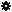﻿ Object Model: PolygonFaceCollection.NeighborVertices

PolygonFaceCollection.NeighborVertices

## PolygonFaceCollection.NeighborVerticesv3.0

### Description

Returns a collection of Vertex objects for all vertex neighbors within a given distance.

### C# Syntax

 ```VertexCollection PolygonFaceCollection.NeighborVertices( Int32 in_lDistance ); ```

### Scripting Syntax

 ```oReturn = PolygonFaceCollection.NeighborVertices( [Distance] ); ```

### Parameters

Parameter Type Description
Distance Integer Distance is an integer value representing the degree of neighborhood (eg: degree=2 for a polygon means its adjacent vertices plus the adjacent vertices of the adjacent polygons)

Default Value: 1

### Examples

#### VBScript Example

 ```set oCube = ActiveSceneRoot.AddGeometry( "Cube", "MeshSurface" ) set oGeometry = oCube.ActivePrimitive.Geometry set oVertices = oGeometry.Vertices set oNeighborPolygons = oVertices(0).NeighborPolygons(1) set oNeighbors = oNeighborPolygons.NeighborVertices( 1 ) str = "Vertices neighbors of this PolygonFaceCollection are :" for each vertex in oNeighbors str = str & " " & vertex.index next logmessage str ```